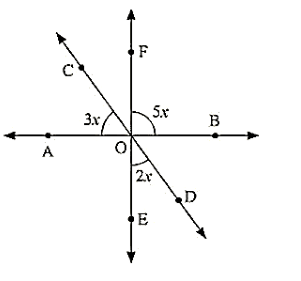# In the below fig. find the value of x?

Question:

In the below fig. find the value of x?Solution:

Vertically opposite angles are equal

AOE = BOF = 5x

Linear pair

∠COA + ∠AOE + ∠EOD = 180°

3x + 5x + 2x = 180

10x = 180

x = 180/10

x = 18

Hence, the value of x = 18°